# Project Euler 203

Squarefree Binomial Coefficients. Building Pascals triangle to 51 rows (look it up – its a bloody nightmare to write out here): Locate all distinct numbers in that triangle, that does not divide by a square of a prime.

OK. First step is to get all the numbers in 51 rows of Pascals triangle.

R has a built in function, choose(i,j) that returns the number for row i, position j.

We can use that to iterate through all the possible positions in a 51 row triangle:

``````numbers <- 1
for(i in 0:50){
for(j in 0:i){
numbers <- c(numbers, choose(i,j))
}
}
``````

Next step is to make sure that we only have unique, distinct, values:

``````numbers <- unique(numbers)
``````

We now need to divide each number by the square of a prime. My first instinct was to generate all primes smaller than the squareroot of the largest number in numbers.

That would be all primes lower than 11,243,247.

I would then square all those primes, and see if one of them divided neatly into the numbers in the triangel.

Thats an awfull lot of primes.

Much easier would be to note, that if the square of a prime divides neatly into a number, then the prime does as well. And is in fact a prime factor in that number.

And since we have a nice library that makes it easy to get the primefactors, thats the way to do it.

``````library(numbers)
library(purrr)

res <- numbers %>%

``````

Passing the numbers vector to the discard function. Discard the element, if the element, modulo the primefactors in that element squared, has one (or more) results that are equal to 0.

The answer is the sum of the elements that are left. Plus 1, since 1 is discarded by the function. factors(1) – for some reason – returns 1.

Nice and simple really.

# Corresponding value to a max-value

One of our users need to find the max-value of a variable. He also needs to find the corresponding value in another variable.
As in – the maximum value in column A is in row 42. What is the value in column B, row 42.

And of course we need to do it for several groups.

Let us begin by making a dataset. Four groups in id,

``````library(tidyverse)
id <- 1:3
val <- c(10,20)
kor <- c("a", "b", "c")

example <- expand.grid(id,val) %>%
as_tibble() %>%
arrange(Var1) %>%
cbind(kor, stringsAsFactors=F) %>%
rename(group=Var1, value=Var2, corr = kor)

example
``````
```##   group value corr
## 1     1    10    a
## 2     1    20    b
## 3     2    10    c
## 4     2    20    a
## 5     3    10    b
## 6     3    20    c
```

We have six observations, divided into three groups. They all have a value, and a letter in “corr” that is the corresponding value we are interested in.

So. In group 1 we should find the maximum value 20, and the corresponding value “b”.
In group 2 the max value is stil 20, but the corresponding value we are looking for is “a”.
And in group 3 the max value is yet again 20, but the corresponding value is now “c”.

How to do that?

``````example %>%
group_by(group) %>%
mutate(max=max(value)) %>%
mutate(max_corr=corr[(value==max)]) %>%
ungroup()
``````
```## # A tibble: 6 x 5
##   group value corr    max max_corr
##   <int> <dbl> <chr> <dbl> <chr>
## 1     1   10. a       20. b
## 2     1   20. b       20. b
## 3     2   10. c       20. a
## 4     2   20. a       20. a
## 5     3   10. b       20. c
## 6     3   20. c       20. c
```

The maximum value for all groups is 20. And the corresponding value to that in the groups is b, a and c respectively.

Isn’t there an easier solution using summarise function? Probably. But our user needs to do this for a lot of variables. And their names have nothing in common.

# Number of words in word

Simple: There is a count at the bottom left.

If you want to insert the word count in the word document:

• Choose “Insert” in the ribbon.
• Click “Quick Parts”, or in danish “Genvejselementer”
• Click “Fields” (Felter)
• Locate “NumWords”, and click on that.

And you’re done!

# Openstreetmap data – for Florence

Not that advanced, but I wanted to play around a bit with plotting the raw data from Openstreetmap.

We’re going to Florence this fall. It’s been five years since we last visited the fair city, that has played such an important role in western history.

Openstreetmaps is, as the name implies, open.

I’m going to need some libraries

``````#library(OpenStreetMap)
library(osmar)
library(ggplot2)
library(broom)
library(geosphere)
library(dplyr)
``````

osmar provides functions to interact with Openstreetmap. ggplot2 is used for the plots, broom for making some objects tidy and dplyr for manipulating data.

Getting the raw data, requires me to define a boundary box, encompassing the part of Florence I would like to work with. Looking at https://www.openstreetmap.org/export#map=13/43.7715/11.2717, I choose these coordinates:

``````top <- 43.7770
bottom <- 43.7642
left <- 11.2443
right <- 11.2661
``````

After that, I can define the bounding box, tell the osmar functions at what URL we can find the relevant API (this is just the default). And then I can retrieve the data via get_osm(). I immediately save it to disc. This takes some time to download, and there is no reason to do that more than once.

``````box <- corner_bbox(left, bottom, right, top)
src <- osmsource_api(url = "https://api.openstreetmap.org/api/0.6/")
florence <- get_osm(box, source=src)
saveRDS(florence, "florence.rda")
``````

Lets begin by making a quick plot:

``````plot(florence, xlim=c(left,right),ylim=c(bottom,top) )
``````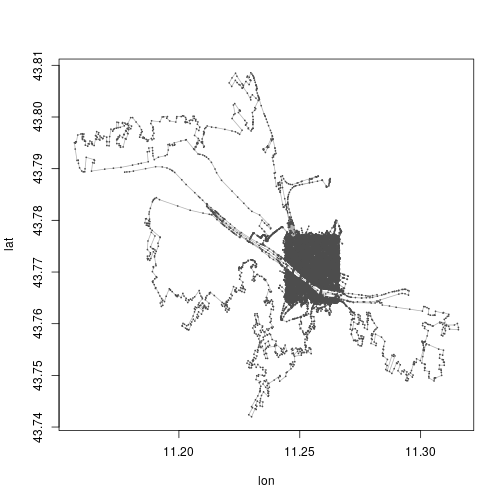Note that what we get a plot of, is, among other things, of all lines that are partly in the box. If a line extends beyond the box, we get it as well.

Looking at the data:

``````summary(florence\$ways)
``````
```## osmar\$ways object
## 6707 ways, 9689 tags, 59052 refs
##
## ..\$attrs data.frame:
##     id, visible, timestamp, version, changeset, user, uid
## ..\$tags data.frame:
##     id, k, v
## ..\$refs data.frame:
##     id, ref
##
## Key-Value contingency table:
##         Key         Value Freq
## 1  building           yes 4157
## 2    oneway           yes  456
## 3   highway    pedestrian  335
## 4   highway   residential  317
## 5   bicycle           yes  316
## 6       psv           yes  122
## 7   highway  unclassified  108
## 8   highway       footway  101
## 9   barrier          wall   98
## 10  surface paving_stones   87
```

I would like to plot the roads and buildings. For some reason there are a lot of highways, of a kind I would probably not call highways.

Anyway, lets make a list of tags. tags() finds the elements that have a key in the tag_list, way finds the lines that are represented by these elements, and find, finds the ID of the objects in “florence” matching this.
find_down() finds all the elements related to these id’s. And finally we take the subset of the large florence data-set, which have id’s matching the id’s we have in from before.

``````tag_list <- c("highway", "bicycle", "oneway", "building")
dat <- find(florence, way(tags(k %in% tag_list)))
dat <- find_down(florence, way(dat))
dat <- subset(florence, ids = dat)
``````

Now, in a couple of lines, I’m gonna tidy the data. That removes the information of the type of line. As I would like to be able to color highways differently from buildings, I need to keep the information.
Saving the key-part of the tags, and the id:

``````types <- data.frame(dat\$ways\$tags\$k, dat\$ways\$tags\$id)
names(types) <- c("type", "id")
``````

This gives me all the key-parts of all the tags. And I’m only interested in a subset of them:

``````types <- types %>%
filter(type %in% tag_list)

types\$id <- as.character(types\$id)
``````

Next as_sp() converts the osmar object to a spatial object (just taking the lines):

``````dat <- as_sp(dat, "lines")
``````

tidy (from the library broom), converts it to a tidy tibble

``````dat <- tidy(dat)
``````

That tibble is missing the types – those are added.

``````new_df <- left_join(dat, types, by="id")
``````

And now we can plot:

``````new_df %>%
ggplot(aes(x=long, y=lat, group=group)) +
geom_path(aes(color=type)) +
scale_color_brewer() +
xlim(left,right) +
ylim(bottom,top) +
theme_void() +
theme(legend.position="none")
``````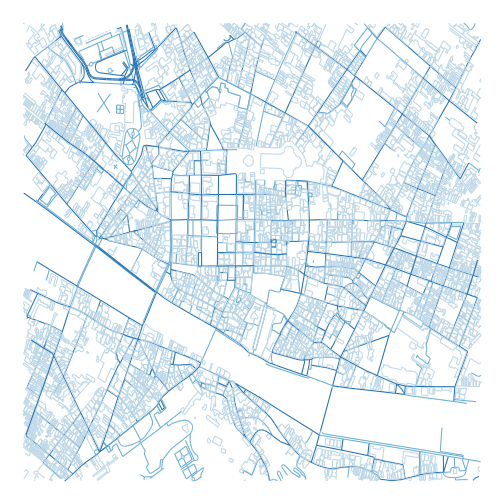Nice.

# Euler problem 62

The cube, 41063625 (3453), can be permuted to produce two other cubes: 56623104 (3843) and 66430125 (4053). In fact, 41063625 is the smallest cube which has exactly three permutations of its digits which are also cube.

Find the smallest cube for which exactly five permutations of its digits are cube.

Alright. I need to find five cubes, that are permutations of the same digits.

How to check if two numbers are permutations of each other?

We can generate the largest permutation of a given number. If the largest permutation of two numbers are identical, the two numbers are permutations of each other.

So I need a function, that returns the largest permutation of a number. It would be nice, if that function was vectorized.

``````max_perm <- function(t){
require(magrittr)
options(scipen=5)
t %>%
as.character() %>%
str_split("") %>%
lapply(sort, decreasing=TRUE) %>%
lapply(paste0, collapse="") %>%
unlist() %>%
as.double()
}
``````

Convert the input to character. Split at “”. That returns a list with vectors containing the individual digits of the input. lapply sorts the individual vectors in the list in decreasing order. Then lapply pastes the elements in each vector together with paste0 and “” as the separator. Then it is unlisted, and returned as numeric.

What is worth noting is a thing I was struggling with for far too long. R likes to write numbers in scientific notation. As in “1e+06”. I have not studied the phenomenon in detail. But options(scipen=5) solves the problem. It is the “penalty” used to decide when a number should be written in scientific notation. Unless I change that (trial and error, but it should be larger than whatever is default), as.character(1000000) will return “1e+06”. And the permutations of “1” “e” “+” “0” “6” are not terribly useful in this context.

I’m hazarding a guess that I don’t need to handle cubes of values of more than four digits.

Beginning with a vector of all numbers from 1 to 9999, I convert it to a dataframe. I transmute the first column to a column with the name x.
Then I mutate a second column, cube, into existence, and calculate it as the cube of the x-value. A third column, max_cube, is mutated with the result from my max_perm function above. And tha column is immediately used to group the data, so I get date grouped by identical maximum values of the permutations. I filter on the count of those groups, and only keep the groups that contain 5 elements. Then I ungroup it, and select just the cube column.

I now have a data frame with a single column containing 10 values. They are all cubes, five of them are permutations of each other. The other five are also permutaions of each other. And now I just have to take the smallest of them.

``````result <- 1:9999 %>%
as.double() %>%
as.data.frame() %>%
transmute(., x = .) %>%
mutate(cube = x**3) %>%
mutate(max_cube = max_perm(cube)) %>%
group_by(max_cube) %>%
filter(n()==5) %>%
ungroup() %>%
select(cube) %>%
min()
``````

Before I print the result, so I can enter it into Project Euler, I set options(digits=17).

Done! A good exercise. And a valuable lesson in the importance of the options in R.

# Waffle charts

A rather popular chart type. Not really my favorite, but I can see how it makes things easier to understand for people who are not used to read and understand charts. The reason for my less than favourable view on waffle charts are probably linked to its overuse in meaningless infographics.

A waffle chart is a grid with squares/cells/icons/whatever, where each cell represents a number of something.

Lets make an example:

``````library(ggplot2)
library(waffle)
``````
``````vec <- c(`Category 1 (10)`= 10 , `Category 2 (20)`= 20,
`Category 3 (25)`= 24, `Category 4 (16)` = 16)

waffle(vec/2, rows=3, size=0.1,
colors=c("#c7d4b6", "#a3aabd", "#a0d0de", "#97b5cf"),
title="Four different categories of something",
xlab="1 square = 2 somethings")
``````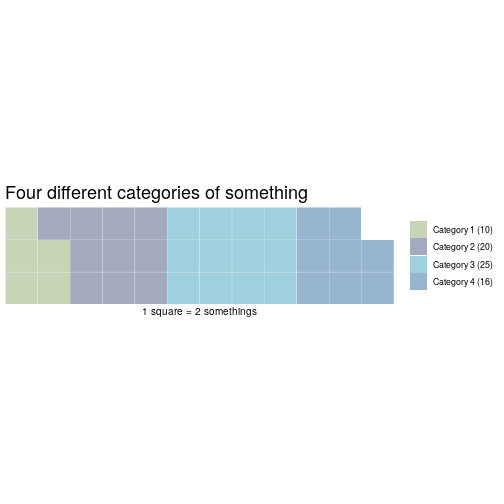One annoyance: waffle wants you to spell colours wrong.

waffle takes a named vector of values, rows sets the number of rows of blocks. Default is 10.

One standard way, is to show a 10×10 grid, where each cell represents 1% of the total:

``````waffle(vec/sum(vec)*100)
``````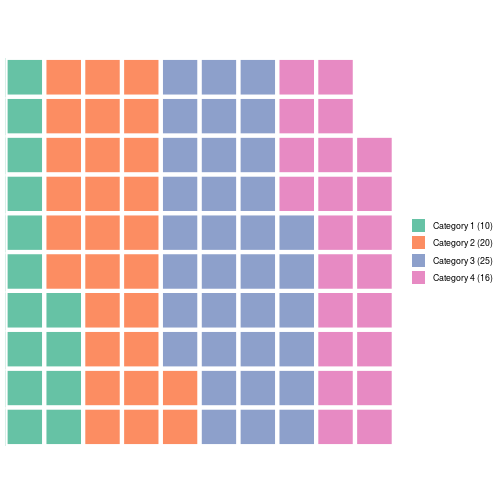Bloody annoying – waffle rounds the values of the vector, leading to only 98 squares. So you have to manipulate your vector to get to 100. Well, actually it is probably a minor annoyance.

What if you want something else than coloured squares?

The arguments “use_glyph” and “glyph_size” makes that possible.
First, we’ll need the library extrafont

``````library(extrafont)
``````

We’ll also need to have the “awesomefonts” installed. It can be downloaded from:

http://maxcdn.bootstrapcdn.com/font-awesome/4.3.0/fonts/fontawesome-webfont.ttf

This should be easier if you are on a desktop machine. As I’m running this through my own installation of RStudio on a remote server, it was a bit more difficult.

I needed to place the “fontawesome.ttf” file in the “/usr/share/fonts/truetype/fontawesome” directory.

Then, running R as superuser on the commandline, I imported the extrafont library, and then ran “font_import()”.

But then it worked!

There is now a long list of 593 different icons, that can be used. If you want a list, just run fa_list().

And now, we can make a waffle chart with the glyph of our choice.

``````waffle(vec/2, rows=4, use_glyph = "wifi")
``````
```## Warning: Removed 1 rows containing missing values (geom_text).
```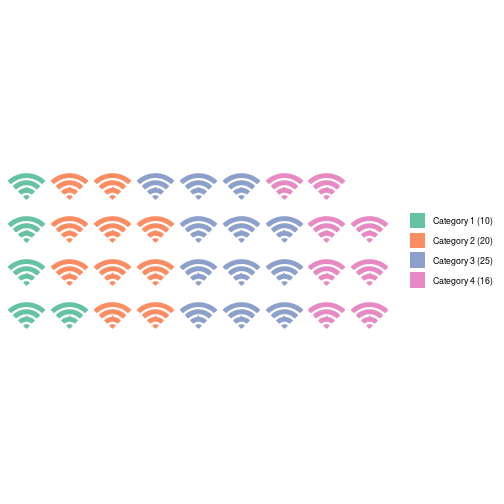We can change the colours:

``````library(RColorBrewer)
waffle(vec/2, rows=4, use_glyph = "wifi", colors=brewer.pal(4,"Set1"))
``````
```## Warning: Removed 1 rows containing missing values (geom_text).
```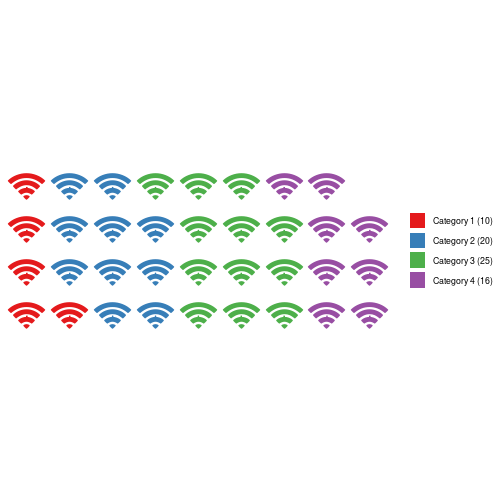``````waffle(vec/2, rows=4, use_glyph = "wifi", colors=brewer.pal(6,"Set1"), glyph_size=5)
``````
```## Warning: Removed 1 rows containing missing values (geom_text).
```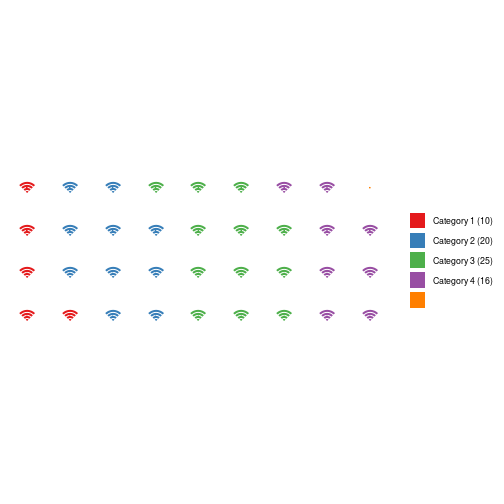But that does not look very good.

waffle is based on ggplot, so we have access to the full range of functionality. But not all of them are going to look good in this context:

``````waffle(vec/2, rows=4, use_glyph = "wifi", colors=brewer.pal(4,"Set1")) +
geom_label(label="42", size = 3)
``````
```## Warning: Removed 1 rows containing missing values (geom_text).
```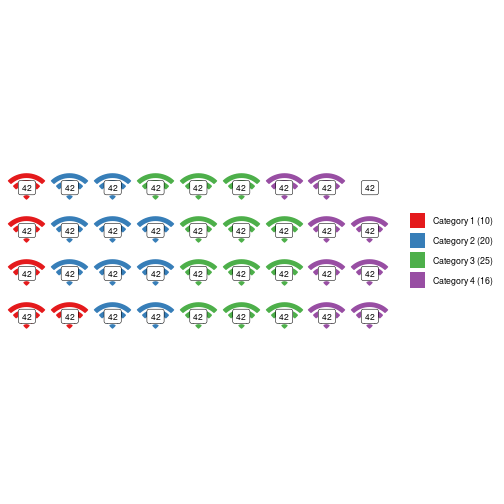If we install a font that supports it, we even get access to the large number of UTF-8 glyphs. Here is a favorite of mine:

``````waffle(vec/2, rows=4, colors=brewer.pal(4,"Set1")) +
geom_label(label=sprintf("\U1F427"), size = 8)
``````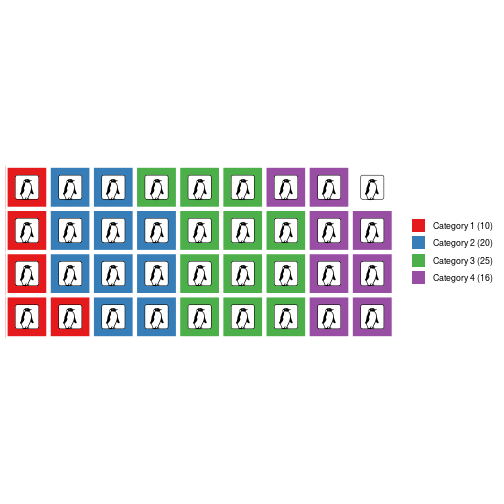Which of course requires you to have a font on your computer that supports penguins.

Here is one:
http://users.teilar.gr/~g1951d/Symbola.zip

Udgivet i R

# Get the font color of a cell in Excel

People do weird and wonderful things in Excel.

Other people then have to pull out the data from those spreadsheets.

“Other people”  tend to spend a lot of time crying into their coffee.

At the moment, I am trying to pull out data of a spreadsheet, where “something” can have a value of 1, 2 or 3. That is of course marked by an “x” in a cell. I need to convert that x to a number.

That is rather simple. What is not so simple, is that there can be two x’es. One, in black, to denote the current state of affairs. And a second x, in red, to denote what a future, state is wanted to be.

So – I need a way to get the color of an x. VBA can do that:

Function GetColour(ByVal Target As Range) As Single
Application.Volatile
GetColour = Target.Font.Color
End Function

And if I need a logical test:

Function IsBlack(ByVal Target As Range) As Boolean
Application.Volatile
If Target.Font.Color = 0 Then
IsBlack = True
Else
IsBlack = False
End If
End Function

# Project Euler 39

We’re looking at Pythagorean triplets, that is equations where a, b and c are integers, and:

a2 + b2 = c2

The triangle defined by a,b,c has a perimeter.

The triplet 20,48,52 fulfills the equation, 202 + 482 = 522. And the perimeter of the triangle is 20 + 48 + 52 = 120

Which perimeter p, smaller than 1000, has the most solutions?

So, we have two equations:

a2 + b2 = c2

p = a + b + c

We can write

c = p – a – b

And substitute that into the first equation:

a2 + b2 = (p – a -b)2

Expanding the paranthesis:

a2 + b2 = p2 – ap – bp – ap + a2 + ab – bp + ab + b2

Cancelling:

0 = p2 – 2ap – 2bp + 2ab

Isolating b:

0 = p2 – 2ap – b(2p – 2a)

b(2p – 2a) = p2 – 2ap

b = (p2 – 2ap)/(2p – 2a)

So. For a given value of p, we can run through all possible values of a and get b. If b is integer, we have a solution that satisfies the constraints.

The smallest value of a we need to check is 1. But what is the largest value of a for a given value of p?

We can see from the pythagorean equation, that a =< b < c. a might be larger than b, but we can then just switch a and b. So it holds. What follows from that, is that a =< p/3.

What else? If a and b are both even, a2 and b2 are also even, then c2 is even, and then c is even, and therefore p = a + b + c is also even.

If a and b are both uneven, a2 and b2 are also uneven, and c2 is then even. c is then even. And therefore p = a + b + c must be even.

If either a or b are uneven, either a2 or b2 is uneven. Then c2 is uneven, and c is then uneven. Therefore p = a + b + c must be even.

So. I only need to check even values of p. That halves the number of values to check.

Allright, time to write some code:

``````current_best_number_of_solutions <- 0

for(p in seq(2,1000,by=2)){
solutions_for_current_p <- 0
for(a in 1:ceiling(p/3)){
if(!(p**2-2*a*p)%%(2*p-2*a)){
solutions_for_current_p <- solutions_for_current_p + 1
}
}
if(solutions_for_current_p > current_best_number_of_solutions){
current_best_p <- p
current_best_number_of_solutions <- solutions_for_current_p
}
}

``````

current_best_number_of_solutions is initialized to 0.

For every p from 2 to 1000, in steps of 2 (only checking even values of p), I set the number of solutions_for_current_p to 0.

For every value a from 1 to p/3 – rounded to to an integer: If !(p2-2*a*p)%%(2*p-2*a) is true, that is, if the remainder of (p2-2*a*p)/(2*p-2*a) is 0, I increment the solutions_for_current_p.

After running through all possible values of a for the value of p we have reached in the for-loop:

If the number of solutions for this value of p is larger, than the previous current_best_number_of_solutions, we have found a value of p that has a higher number of solutions than any previous value of p we have examined. In that case, set the current_best_p to the current value of p. And the current_best_number_of_solutions to the number of solutions we have found for the value of p.

If not, dont change anything, reset solutions_for_current_p and check a new value of p.

# Crimes against graphs

Crime is a bad thing. No doubt about it. And one of the main topics in todays debate climate is – “those ‘orrible immigrants are very criminal. Look at these numbers, they prove it!”. Usually written with caps-lock engaged.

Well. Maybe they are, and maybe they do. But if you want to use statistics to prove it – pretty please, do not obfuscate the numbers.

This is an example. A blog post from one of the more notable danish newspapers. In the US it would be regarded as communist, in the rest of the world we would think of it as relatively conservative.

https://kulturkamp.blogs.berlingske.dk/2018/08/17/anmeldte-voldtaegter-og-voldsforbrydelser-er-paa-det-hoejeste-nogensinde/

The claim is, that the number of reported rapes and other violent crimes in Denmark, are the highest ever. That is because of the increasing numbers of immigrants in Denmark, especially muslims. Use Google translate if you want the details.

Again, that claim might be true. But the graphs in the post, that supposedly documents the claim, are misleading. To say the least.

First – the numbers come from the Danish Statistical Bureau. They have a disclaimer, telling us that changes to the danish penal code, means that a number of sexual offenses have been reclassified as violent crimes since 2013. If the number of violent crimes suddenly includes crimes that did not use to be classified as violent crimes, that number will increase. Not much of a surprise. Yes, the post asks why the numbers are still increasing after that reclassification. One should expect them to level off. And again the post may have a valid point. I don’t know. But what I do know, is that the graphs are misleading.

Heres why. The y-axis has been cut of. Lets recreate the graphs, and take a look.

There are two graphs. The first shows the number of reported cases of rape from 1995 until today.

The second shows the total number of reported cases of violent crimes in the same period. Both sets of data comes from http://www.statistikbanken.dk/.

We’re going to need some libraries:

``````library(ggplot2)
library(gridExtra)
``````

Lets begin by pulling the data.

There might be better ways, but I’ve simply downloaded the data. Two files:

``````violence <- read.csv("tab1.csv", sep=";", skip=3, header=F)
violence <- violence[1:(nrow(violence)-7),]
rape <- rape[1:(nrow(rape)-7),]
``````

The last seven lines are the notes about changes in which cases are counted in this statistics. I think that is a pretty important point, but they are difficult to plot.

The graph for rape, as presented in the post, and with a more sensible y-axis:

``````post <- ggplot(rape, aes(x=V1, y=V2)) +
geom_line(group=1) +
scale_x_discrete(breaks = rape\$V1[seq(1, length(rape\$V1), by = 20)]) +
theme_classic()

nice <- post + ylim(0,max(rape\$V2))
grid.arrange(post, nice, ncol=2)
``````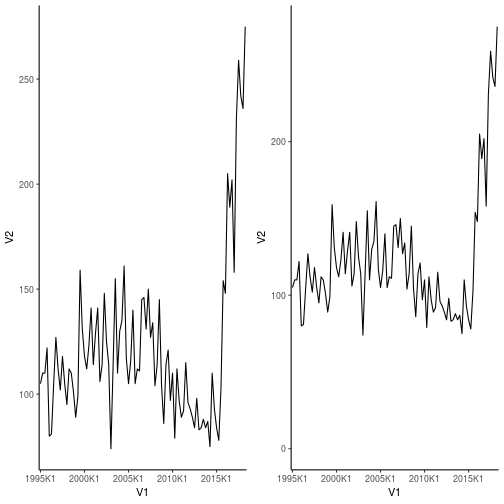And the one for violent crimes in general, again with the original on the left, and the better on the right:

``````post <- ggplot(violence, aes(x=V1, y=V2)) +
geom_line(group=1) +
scale_x_discrete(breaks = violence\$V1[seq(1, length(violence\$V1), by = 20)]) +
theme_classic()

nice <- post + ylim(0,max(violence\$V2))
grid.arrange(post, nice, ncol=2)
``````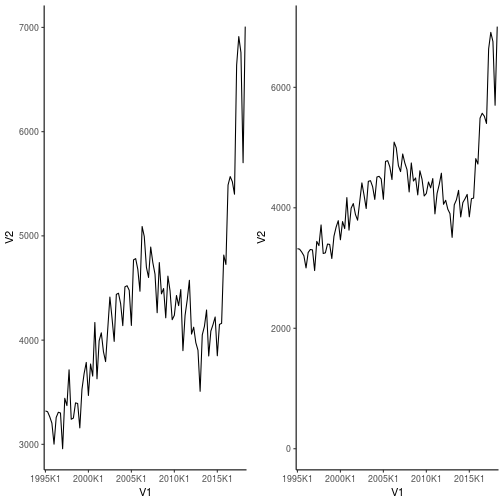So, still, some pretty scary increases. And the change in what is counted should give an increase. But that increase should level off, which it does not. Clearly something is not as it should be. But lets be honest, the graphs on the right are not quite as scary as the ones on the left.

Also – that change in what is counted as sexual assaults – it can explain the initial increase, but then it should level off. That is a fair point. However, there were other things that changed in the period. #metoo for example. I think it would be reasonable to expect that a lot of cases that used to be brushed of as not very important, are now finally being reported. The numbers might actually have leveled off without #metoo.

Anyway, my point is, that if you want to use graphs to support your claims, do NOT cut off the y-axis to make them look more convincing.